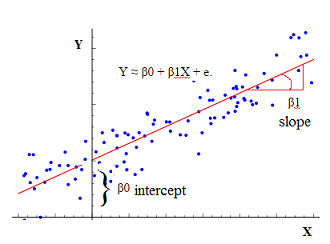# Statistics - (No Predictor|Mean|Null) Model

The simplest prediction in a regression that you can imagine is:

• using the mean of the target ($\bar{Y}$ ) as prediction
• ie choosing a slope of 0

The mean model is also known as the “No Model”.

With this model, the error RSS is equal to TSS.

The null model has no predictors. It just contains one intercept where the intercept is the mean of Y.

Recommended PagesStatistics - (Total) Sum of the square (TSS|SS)

SS or TSS is the sum of the square deviation scores from the mean for one variable. It's the error calculation of the no predictor model (The basis model).Statistics - (Univariate|Simple|Basic) Linear Regression

A Simple Linear regression is a linear regression with only one predictor variable (X). Correlation demonstrates the relationship between two variables whereas a simple regression provides an equation...Statistics - Best Subset Selection Regression

The most direct approach in order to generate a set of model for the feature selection approach is called all subsets or best subsets regression. We compute the least squares t for all possible subsets...Statistics - Forward and Backward Stepwise (Selection|Regression)

In statistics, stepwise regression includes regression models in which the choice of predictive variables is carried out by an automatic procedure. Stepwise methods have the same ideas as best subset...